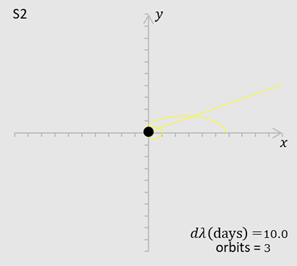## Tuesday, 10 November 2020

### Plotting geodesics of Schwarzschild

I did some experiments with trying to plot solutions to the geodesics of Schwarzschild. They might give orbits of stars round the black hole Sagittarius A* at the centre of our galaxy. The geodesic equations become three simultaneous second order differential equations which give what I call the chugger equations below:$t$ is the coordinate time and $r,\theta$ are the usual plane polar coordinates. We are only considering curves in one plane. A prime indicates a derivative with respect to $\lambda$ which is the affine parameter from the geodesic equation and could be proper time. $G$ is old Newton's gravitational constant, $M$ is the mass of Sagittarius A* and $c$ is the speed of light.We start with some initial values of $t,t^\prime,r,r^\prime,\theta,\theta^\prime$ and select some $d\lambda$ . Then we use those to get new values of  $t,t^\prime,r,r^\prime,\theta,\theta^\prime$ from the chugger equations again and again and again and put them in successive rows of a spreadsheet. The spreadsheet then uses the values of $r,\theta$ to plot the curve. One of the stars orbiting Sagittarius A* is S2 and we use that as our example. It completes an orbit about every 16 years. The first image was my first attempt. The shape is good (it follows the top part of the Newtonian ellipse) until it gets pretty close to the black hole. The second image shows a close up of the same curve near the black hole.

The dashed line is the ellipse that S2 would follow according to Newton's equations.

If I 'manually' decreased $d\lambda$ when near the black hole and then increased it again as S2 departed I could get the third image which shows the last leg of the approximation. To be able to do that conveniently I had to program the chugger equations in VBA. To do 1,000 iterations takes about three minutes.

Finally I did all the calculations in Excel adjusting $d\lambda$ as it went along and got the fourth image from a 20,000 row spreadsheet. The calculation time is about one second. It was the best so far but still not good enough. The furthest distance (apsis) of S2 from the black hole decreases by 4% - it should be the same. However the furthest distance did advance by 0.0012 radians. I calculated (with help from Carroll) that it should be 0.0035 radians.

For a bit of fun I also made an artist's impression of precession of the perihelion. It's simply done from the exact solution to Newton's equations which is an ellipse.

Further material including spreadsheets and VBA at